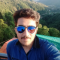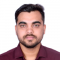## How to Create a Candlestick Chart in Matplotlib?Updated on 18-Apr-2023 14:56:30
Candlestick charts are a popular way to visualize stock market data. They show the opening, closing, high, and low prices of a stock or security for a given time period. A candlestick chart consists of a series of vertical bars or "candlesticks", where each candlestick represents one time period. The top and bottom of each candlestick represent the highest and lowest prices traded during that period, while the body of the candlestick represents the opening and closing prices. In this tutorial, we will explore codes where we will use Matplotlib, a popular data visualization library in Python, to create a ... Read More

## How to Annotate Matplotlib Scatter Plots?Updated on 24-Mar-2023 15:14:36
Introduction Scatter plots are an essential tool for illustrating the connection between two continuous variables. They help us identify potential anomalies, patterns, and trends in the data. Yet, scatter charts can also be hard to interpret when there are numerous data points. If comments are made, some points of interest in a scatter plot could be easier to observe and understand. In order to make Matplotlib scatter plots more understandable, this article will examine how to annotate them. Syntax ax.annotate(text, xy, xytext=None, arrowprops=None, **kwargs) text − Text to be displayed in the annotation. xy − (x, y) coordinates ... Read More

## How to Annotate Bars in Grouped Barplot in Python?Updated on 24-Mar-2023 15:13:21
Introduction As data visualization becomes an integral part of every data analysis project, bar plots serve as a great tool to represent categorical data. Grouped bar plots in particular are useful when we want to compare multiple groups side-by-side. Syntax and Use Cases Annotations can be added to a bar plot to provide additional information or clarification to the data being presented. The annotation function of matplotlib can be used to add these annotations to each bar. The function takes the following parameters − text − The text to be displayed in the annotation. xy − The point ... Read More

## How To Annotate Bars in Bar Plot with Matplotlib in Python?Updated on 24-Mar-2023 15:04:43
Introduction Bar plots are a common sort of chart used in data visualization. They are a go-to choice for many data scientists since they are easy to produce and comprehend. Bar charts, however, might fall short when we need to visualize additional information. Annotations are useful in this situation. In bar plots, annotations may be used in order to better comprehend the data. Syntax and Usage Use Matplotlib's annotate() function. The method accepts a number of inputs, such as the text to annotate, where the annotation should be placed, and several formatting choices including font size, color, and style. The ... Read More

## How to animate an object using the Arcade module?Updated on 24-Mar-2023 15:03:18
Introduction Python's Arcade module allows users to build interactive animations. It has simple and straightforward documentation for making interactive games, and its object-oriented architecture makes working with animated objects simple. Wonderful Animations with Arcade Module The Arcade module in Python is a Python library for creating 2D video games and can be easily installed by pip installing the arcade package. In order to use Arcade in your Python project, you need to install the Arcade external dependency by running the command "pip install arcade" in the terminal. Let's look at two fantastic uses for this Python package. Create a ... Read More

## How to Adjust the Number of Ticks in Seaborn Plots?Updated on 24-Mar-2023 14:44:38
Introduction Ticks are tiny symbols that Matplotlib uses to represent the positions of data points on both axes of a plot. They may be positioned to best fit the data range and are used to highlight certain locations on the x and y axes. Usually, ticks may be labeled to indicate the precise values they stand for. In the python package Seaborn, there are two functions, namely, xticks() and yticks() that can be used for adjusting the ticks of a given graph. Syntax To adjust the number of ticks in Seaborn plots, we can use the following syntax − ... Read More

## How to Adjust Marker Size in Matplotlib?Updated on 24-Mar-2023 14:38:06
Introduction In a plot, a marker is a symbol that designates a single data point. Size, color, and shape are just a few of the attributes that may be changed. Markers are commonly used in conjunction with other charting methods to enhance the readability and comprehension of data. With Matplotlib, a wide variety of marker shapes are provided, including circles, squares, triangles, diamonds, and more. It is possible to alter the marker size to draw attention to crucial details or to develop more aesthetically pleasing plots. We'll show you how to alter the marker size in Matplotlib using examples of ... Read More

## How to change the attributes of a networkx / matplotlib graph drawing?Updated on 02-Feb-2022 11:56:11
To change the attributes of a netwrokx/matplotlib graph drawing, we can take the following steps −StepsSet the figure size and adjust the padding between and around the subplots.Initialize a graph with edges, name, or graph attributes.Add the graph's attributes. Add an edge between u and v.Get the edge attributes from the graph.Position the nodes with circles.Draw the graph G with Matplotlib.To display the figure, use show() method.Exampleimport matplotlib.pyplot as plt import networkx as nx plt.rcParams["figure.figsize"] = [7.50, 3.50] plt.rcParams["figure.autolayout"] = True G = nx.Graph() G.add_edge(0, 1, color='r', weight=2) G.add_edge(1, 2, color='g', weight=4) G.add_edge(2, 3, color='b', weight=6) G.add_edge(3, 4, ... Read More

## How to fill an area within a polygon in Python using matplotlib?Updated on 02-Feb-2022 11:47:09
To fill an area within a polygon in Python using matplotlib, we can take the following steps −StepsSet the figure size and adjust the padding between and around the subplots.Create a figure and a set of subplots.Get an instance of a polygon.Get the generic collection of patches with iterable polygons.Add a 'collection' to the axes' collections; return the collection.To display the figure, use show() method.Exampleimport matplotlib.pyplot as plt from matplotlib.collections import PatchCollection from matplotlib.patches import Polygon import numpy as np plt.rcParams["figure.figsize"] = [7.50, 3.50] plt.rcParams["figure.autolayout"] = True fig, ax = plt.subplots(1) polygon = Polygon(np.random.rand(6, 2), closed=True, alpha=1) ... Read More

## How to get data labels on a Seaborn pointplot?Updated on 02-Feb-2022 11:37:50
To get data labels on a Seaborn pointplot, we can take the following steps −StepsSet the figure size and adjust the padding between and around the subplots.Create a dataframe, df, of two-dimensional, size-mutable, potentially heterogeneous tabular data.Create a pointplot.Get the axes patches and label; annotate with respective labels.To display the figure, use show() method.Examplefrom matplotlib import pyplot as plt import pandas as pd import seaborn as sns plt.rcParams["figure.figsize"] = [7.50, 3.50] plt.rcParams["figure.autolayout"] = True df = pd.DataFrame({'a': [1, 3, 1, 2, 3, 1]}) ax = sns.pointplot(df["a"],    order=df["a"].value_counts().index) for p, label in zip(ax.patches, df["a"].value_counts().index):    ax.annotate(label, ... Read More